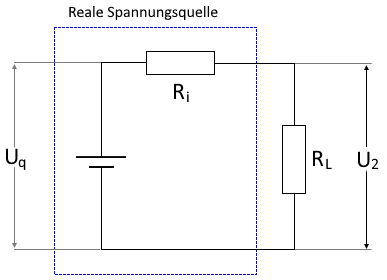# Internal resistance of electrical power sources

Online calculator and formulas for calculating the internal resistance of electrical sources

## Calculate internal resistance

The internal resistance of an electrical source can be calculated by comparing two different load conditions. To do this, measure the open circuit voltage without load. Then the voltage source is loaded with a resistor and the loaded voltage is measured. With the determined data you can calculate the internal resistance of the voltage source on this page.

internal resistance online calculator

 Input Open circuit voltage µV mV V kV Loaded voltage µV mV V kV Load Resistance mΩ Ω kΩ MΩ Decimal places 0 1 2 3 4 6 8 10 Result Internal resistance Current

## Formula for calculating the internal resistance

The internal resistance can be calculated using two load conditions.The current with a loaded power source can be calculated using the following formula:

$$\displaystyle I=\frac{U_2}{R_L}$$

The internal resistance can then be calculated using the current and the voltage difference.

$$\displaystyle R_i=\frac{U_q-U_2}{I}$$

Legend

 $$\displaystyle U_q$$ Source voltage $$\displaystyle U_2$$ Terminal voltage $$\displaystyle R_{i}$$ Internal resistance $$\displaystyle R_{L}$$ Load resistance $$\displaystyle I$$ Current with load resistance $$\displaystyle I_k$$ Short circuit current

More formulas

 Terminal voltage $$\displaystyle U=U_q-(R_i·I_L)$$ Open circuit $$\displaystyle U=U_q$$ Short circuit $$\displaystyle I_k=\frac{ U_q}{R_i}$$

 Ist diese Seite hilfreich?             Vielen Dank für Ihr Feedback! Wie können wir die Seite verbessern?